# Stochastic power ranger trading system

Stochastic power ranger trading system is a momentum strategy.
Time Frame H1 or higher.
Currency pairs: majors at the H1 time frame.
Stochastic power ranger trading system is based on the concept that a range will form after the market finishes trending. We use the stochastic to give us an indication of a possible range formation. We also rely on the current market momentum to tell us when to go long or short. If the market is moving in an uptrend, we look to go long in the range. The entry is determined by the
oversold region (below level 20) of the stochastic.
If the market is moving in a downtrend, we look to go short in the range. The entry is determined by the overbought region (above level 80) of the stochastic. We use the most recent high and low to determine the possible resistance and support of the range.
This strategy has two profi t targets, and we take the fi rst profi t target within the range. The second profit target.

Rules Stochastic power ranger
We use EUR/USD on the H1 time frame to illustrate a long trade. Here are the steps to execute the Stochastic power ranger strategy for long:
1. Draw an uptrend line based on a series of higher highs and higher lows. (See Figure 1 .)
2. On the stochastic, look for %K and %D to go below level 20 (oversold). (See Figure 2.)
3. Determine the support and resistance of the range. Enter long once the stochastic crosses above level 20.
4. Set the first profi t target at the 75% mark of the range. Set the stop loss at a risk to reward ratio of 1:1, and set the second profit target at risk to reward ratio of 1:2. After calculation, the stop loss must be below the support level. If not, the trade is considered invalid. (See Figur3 .)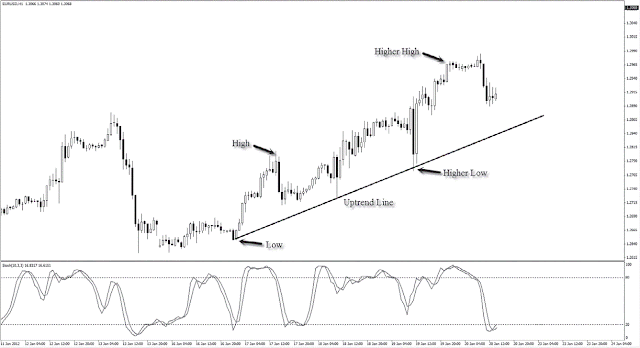12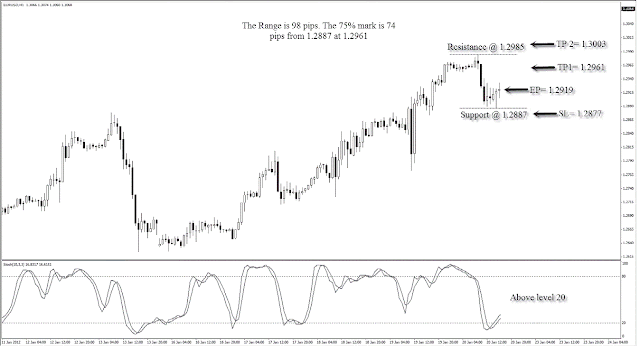34 Stochastic power ranger trading system
from the long example in Figure 4 :
Entry price = 1.2919
Stop loss = 1.2877
Profit target 1 = 1.2961
Profit target 2 = 1.3003
The risk for this trade is 42 pips, and the reward is 84 pips if both targets are hit. The risk to reward ratio is 1:2, which yields a tidy 6% return if we take a 3% risk.

We use AUD/USD on the H1 time frame to illustrate a short trade. Here are
the steps to execute the power ranger strategy for short:
1. Draw a downtrend line based on a series of lower highs and lower lows. (See Figure 5 .)
2. On the stochastic, look for %K and %D to go above level 80 (oversold). (See Figure 6 .)
3. Determine the support and resistance of the range. Enter short once the stochastic goes below level 80.
4. Set the fi rst profit target at the 75% mark of the range. Set the stop loss at a risk to reward ratio of 1:1, and set the second profi t target at risk to reward ratio of 1:2. After calculation, the stop loss must be above the resistance level. If not, the trade is considered invalid. (See Figure 7 .)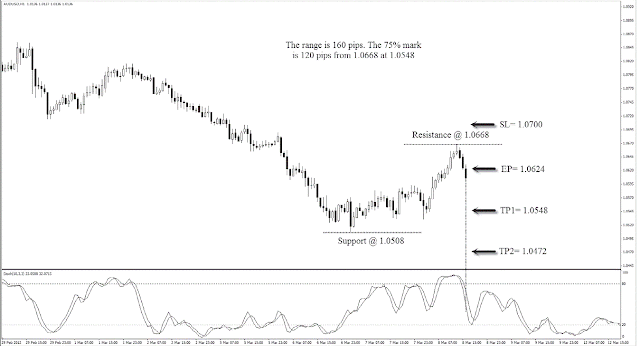56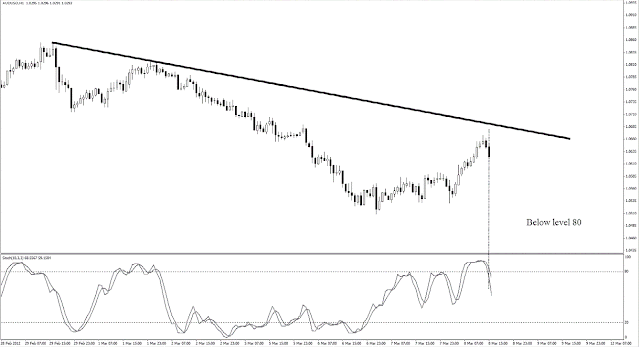7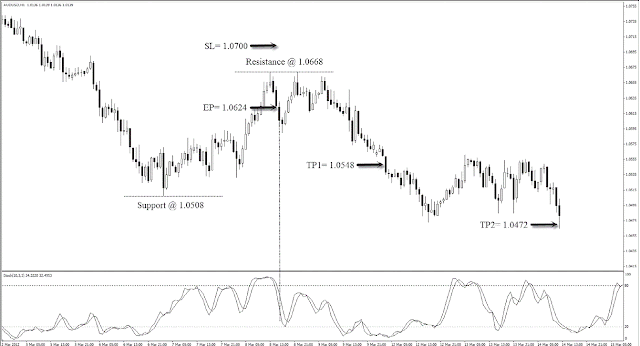8 Stochastic power ranger trading system
From the short example in Figure 8 :
Entry price = 1.0624
Stop loss = 1.0700
Profit target 1 = 1.0548
Profit target 2 = 1.0472
The risk for this trade is 76 pips, and the reward is 152 pips if both targets are hit. The risk to reward ratio is 1:2, which yields a tidy 6% return if we take a 3% risk.